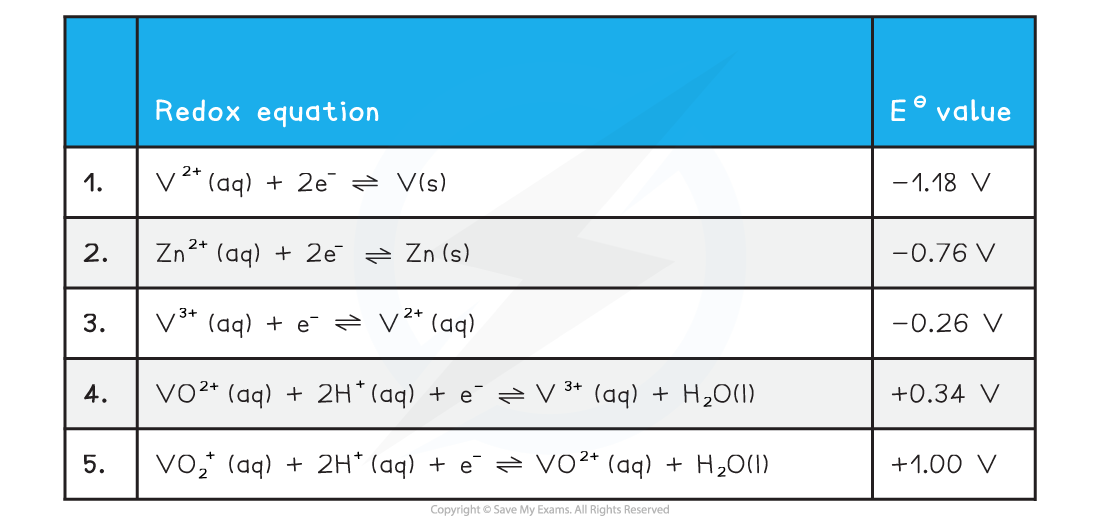# Edexcel A Level Chemistry:复习笔记6.3.1 Vanadium

### Colours & Oxidation States

• Vanadium is a transition metal which has variable oxidation states
• The table below shows the important ones you need to be aware of• Addition of zinc to the vanadium(V) in acidic solution will reduce the vanadium down through each successive oxidation state
•  The colour would successively change from yellow to blue to green to violet
• The ion with the V at oxidation state +5 exists as a solid compound in the form of a VO3- ion
• Usually as NH4VO3 known as ammonium vanadate(V)
• It is a reasonably strong oxidising agent
• Addition of acid to the solid will turn into the yellow solution containing the VO2+ ion.

### Interconversions of Vanadium Ions

• For vanadium we need to consider the following standard electrode potential values
• We will use zinc as our chosen oxidising agent
• The half equations are arranged from high negative EΘ at the top to high positive EΘ at the bottom
• The best reducing agent is the top right species (V2+)
• The best oxidising agent is the bottom left species (VO2+)#### Reduction from +5 to +4

• The two half equations we need to consider are 2 and 5
• Vanadium is reduced from an oxidation number of +5 to +4 in half equation 5
• The EΘ value for half equation 2 is more negative than the EΘ for half equation 5
• Zn is the best reducing agent
• VO2+ is the best oxidising agent
• We can obtain the overall equation by reversing half equation 2 and combining with equation 5
• When adding half equations remember to multiply them so each have the same number of electrons

2VO2+ (aq) + 4H+ (aq) + Zn (s) → 2VO2+ (aq) + Zn2+ (aq) + 2H2O (l)

#### Reduction from +4 to +3

• The two half equations we need to consider are 2 and 4
• Vanadium is reduced from an oxidation number of +4 to +3 in half equation 4
• The EΘ value for half equation 2 is more negative than the EΘ for half equation 5
• Zn is the best reducing agent
• VO2+ is the best oxidising agent
• We can obtain the overall equation by reversing half equation 2 and combining with equation 4
• When adding half equations remember to multiply them so each have the same number of electrons

2VO2+ (aq) + 4H+ (aq) + Zn (s) → 2V3+ (aq) + Zn2+ (aq) + 2H2O (l)

#### Reduction from +3 to +2

• The two half equations we need to consider are 2 and 3
• Vanadium is reduced from an oxidation number of +3 to +2 in half equation 3
• The EΘ value for half equation 2 is more negative than the EΘ for half equation 3
• Zn is the best reducing agent
• V3+ is the best oxidising agent
• We can obtain the overall equation by reversing half equation 2 and combining with equation 3
• When adding half equations remember to multiply them so each have the same number of electrons

2V3+ (aq) + Zn (s) → 2V2+ (aq) + Zn2+ (aq)

#### Reduction from +2 to 0

• The two half equations we need to consider are 1 and 2
• Vanadium is reduced from an oxidation number of +2 to 0 in half equation 1
• The EΘ value for half equation 1 is more negative than the EΘ for half equation 2
• Zn is not electron releasing with respect to V2+
• This means this reaction is not thermodynamically feasible

#### Predicting oxidation reactions

• The same method can be used to predict whether a given oxidising agent will oxidise a vanadium species to one with a higher oxidation number

#### Exam Tip

It is important to not get confused between the two oxo ions of vanadium VO2+ and VO2+